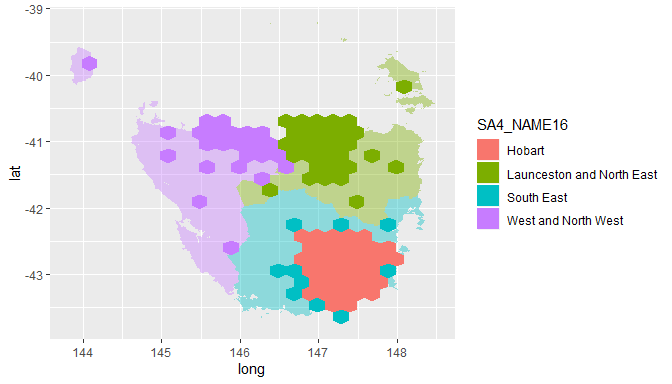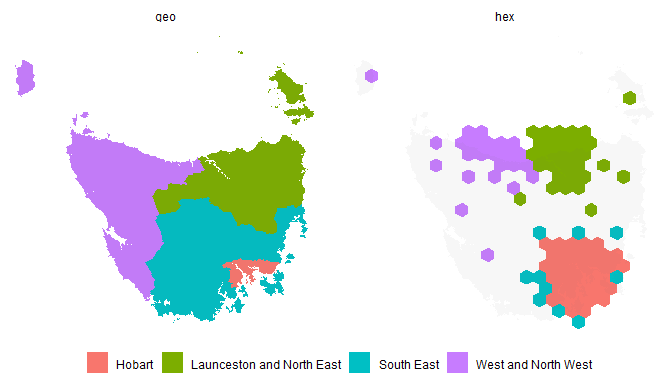# Tasmania

#### 2019-08-20

#remotes::install_github("srkobakian/sugarbag")
library(sugarbag)
library(dplyr)
library(tidyr)
library(tibble)
library(ggplot2)

## Creating a hexagon map of Tasmania

Tasmania is a state of Australia, it has one large land mass and several smaller islands.

### Data

We will use the Australian Bureau of Statistics’ ESRI shape files to build our map. Provided for convenience on the package website:

https://srkobakian.github.io/sugarbag/articles/abs-data.html

The set has been filtered for only Tasmanian areas. The data set of Tasmanian Statistical Areas at level two has been provided as a package data set, ?tas_sa2.

### Centroids

The function create_centroids finds the central points of the polygons provided as an argument.

centroids <- create_centroids(tas_sa2, sf_id = "SA2_5DIG16")

### Hexagon grid

To tesselate correctly, all the hexagons must be evenly spaced. This function creates a grid of possible locations for the polygons.

grid <- create_grid(centroids = centroids, hex_size = 0.2, buffer_dist = 1.2)

### Allocate areas

Each polygon centroid will be allocated to the closest available hexagon grid point. The capital cities data set will be used to preserve neighbourly relationships.

hex_allocated <- allocate(centroids = centroids,
sf_id = "SA2_5DIG16",
hex_grid = grid,
hex_size = 0.2, # same size used in create_grid
hex_filter = 10,
focal_points = capital_cities,
width = 30, verbose = TRUE) # same column used in create_centroids
h1 <- hex_allocated %>%
fortify_hexagon(hex_size = 0.2, sf_id = "SA2_5DIG16") %>%
left_join(., tas_sa2) %>% mutate(poly_type = "hex")

p1 <- fortify_sfc(tas_sa2) %>% mutate(poly_type = "geo")

ggplot(mapping = aes(fill = SA4_NAME16)) +
geom_polygon(data = p1, aes(x=long, lat, group = interaction(SA2_5DIG16,polygon)), alpha = 0.4) +
geom_polygon(data = h1, aes(x=long, lat, group = interaction(SA2_5DIG16)))hex_anim <- h1 %>%
select(SA4_NAME16, SA2_5DIG16,
SA2_NAME16, long, lat, poly_type) %>%
left_join(p1 %>% distinct(SA2_5DIG16, polygon), by = "SA2_5DIG16")
geo_anim <- p1 %>%
select(SA4_NAME16, SA2_5DIG16,
SA2_NAME16, long, lat, polygon, poly_type)
anim_tas <- bind_rows(hex_anim, geo_anim) %>% left_join(homeless)

anim_tas %>%
ggplot(aes(x=long, y=lat, group = interaction(polygon, SA2_5DIG16))) +
geom_polygon(aes(fill = SA4_NAME16)) +
geom_polygon(data = geo_anim %>% select(-poly_type), fill = "grey40", alpha = 0.05) +
coord_equal() +
theme_void() +
guides(fill = guide_legend(title = NULL)) +
theme(legend.position = "bottom") +
facet_wrap(~poly_type)